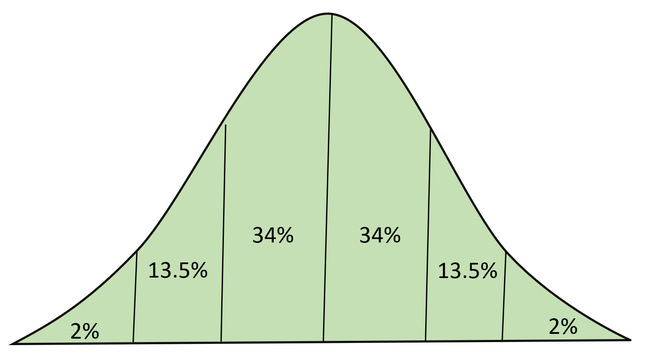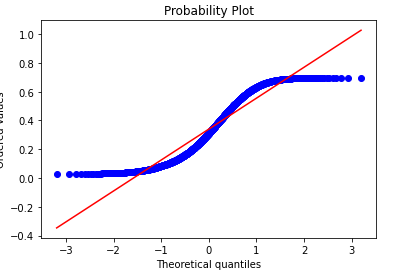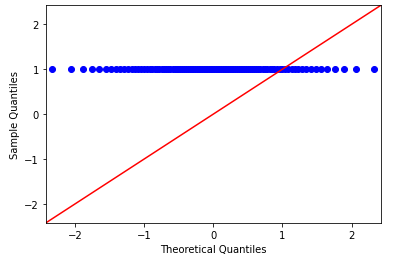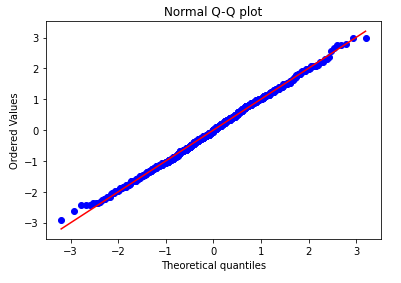# Quantile Quantile plots

• Last Updated : 22 Jan, 2021

The quantile-quantile plot is a graphical method for determining whether two samples of data came from the same population or not. A q-q plot is a plot of the quantiles of the first data set against the quantiles of the second data set. By a quantile, we mean the fraction (or percent) of points below the given value.

For the reference purpose, a 45% line is also plotted, if the samples are from the same population then the points are along this line.

#### Normal Distribution:

The normal distribution (aka Gaussian Distribution/ Bell curve) is a continuous probability distribution representing distribution obtained from the randomly generated real values.Below is the portion of data representing different standard deviationNormal Distribution with Area Under CUrve

#### Usage:

The Quantile-Quantile plot is used for the following purpose:

• Determine whether two samples are from the same population.
• Whether two samples have the same tail
• Whether two samples have the same distribution shape.
• Whether two samples have common location behavior.

#### How to Draw Q-Q plot

• Collect the data for plotting the quantile-quantile plot.
• Sort the data in ascending or descending order.
• Draw a normal distribution curve.
• Find the z-value (cut-off point) for each segment.
• Plot the dataset values against the normalizing cut-off points.

• Since Q-Q plot is like probability plot. So, while comparing two datasets the sample size need not to be equal.
• Since we need to normalize the dataset, so we don’t need to care about the dimensions of values.

#### Types of Q-Q plots

• For Left-tailed distribution: Below is the• For the uniform distribution: Below is the q-q plot distribution for uniform distribution:uniform distribution Q-Q plot

Python code implementation

 # code  import scipy.stats as stats  import numpy as np  import matplotlib.pyplot as plt  %matplotlib inline     n = 2000 observation = np.random.binomial(n, 0.53, size=1000)/n     z = (observation-np.mean(observation))/np.std(observation)     stats.probplot(z, dist="norm", plot=plt)  plt.title("Normal Q-Q plot")  plt.show()

Output:Normal Q-Q plot

My Personal Notes arrow_drop_up
Recommended Articles
Page :